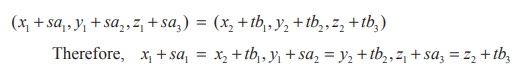Home | | Maths 12th Std | Point of intersection of two straight lines

# Point of intersection of two straight lines

If the equations of straight lines are given in vector form, write them in cartesian form and proceed as above to find the point of intersection.

Point of intersection of two straight lines

Ifare two lines, then every point on the line is of the form (x1 + sa1 , y1 + sa2 , z1 + sa3 ) and (x2 + tb1 , y2 + tb2 , z2 + tb3 ) respectively. If the lines are intersecting, then there must be a common point. So, at the point of intersection, for some values of s and t , we haveBy solving any two of the above three equations, we obtain the values of s and t . If s and t satisfy the remaining equation, the lines are intersecting lines. Otherwise the lines are non-intersecting . Substituting the value of s , (or by substituting the value of t ), we get the point of intersection of two lines.

If the equations of straight lines are given in vector form, write them in cartesian form and proceed as above to find the point of intersection.

### Example 6.33

Find the point of intersection of the lines### Solution

Every point on the line(say) is of the form (2s +1, 3s + 2, 4s + 3) and every point on the line(say) is of the form (5t + 4, 2t +1, t) . So, at the point of intersection, for some values of s and t , we have

(2s +1, 3s + 2, 4s + 3) = (5t + 4, 2t +1, t)

Therefore, 2s − 5t = 3, 3s − 2t = −1 and 4s t = −3 . Solving the first two equations we get t = −1, s = −1 . These values of s and t satisfy the third equation. Therefore, the given lines intersect. Substituting, these values of t or s in the respective points, the point of intersection is (−1, −1, −1) .

Tags : Definition, Solved Example Problems, Solution , 12th Mathematics : UNIT 6 : Applications of Vector Algebra
Study Material, Lecturing Notes, Assignment, Reference, Wiki description explanation, brief detail
12th Mathematics : UNIT 6 : Applications of Vector Algebra : Point of intersection of two straight lines | Definition, Solved Example Problems, Solution